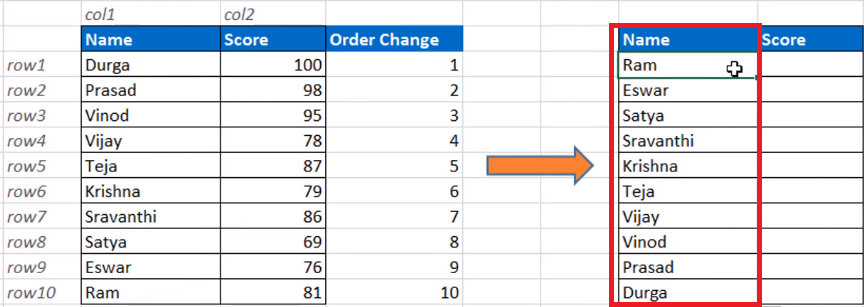top of page
Search
• Dp

# How To Inverse the Data Range in MS Excel || Turn Data Upside Down in Excel

In some situations, you will be required to Inverse the data like the transpose function which does the switching of columns and rows, there is no direct formula like invert to inverse the complete data range upside down in the MS Excel.

Now, in this tutorial, I will show you how to inverse the data range in MS Excel.

In this example consider 10 people and their corresponding scores and let's start inverting the data range.Of course, the simplest method of turning the data upside down is to

1. Add one additional column and name the column as "order change" and

2. Type the sequence of the data range starting from 1 to 10

3. Select the entire data range C5:E14 and select custom sort.

4. From the drop down menu in the sort dialog box, sort by Order change column, in the order largest to smallest5. So you data will get inverse.

Got it friends!!

Other than this,let us see more automated and smarter way by using formulas.

Here I will be using combination of two formulas, Index and Row Functions here.

So, let us understand ROWS function used for.

ROWS function will extract the number of Rows in any selected array.Let's try this by typing formula in cell L5 as = ROWS(C5:C14), which would give the result as "10". If I change the range, the result changes.

Similarly, INDEX function looks at an array and pick the cell value with reference of row number and column number, it is similar to Vlookup function.Let's try this by typing in cell K5 as =INDEX(C5:D14, 1,1), which would give the result as "Durga", which is nothing but our first value of our array.

So, let's start using these two functions and inverse our data range.

1. In Cell H5, I type the formulas as =INDEX(\$C\$5:\$D\$14,ROWS(C5:\$C\$14),1) and press enter to see the result as "RAM" which is the last value of our data range.Here, we have locked the C14 cell and we drag this formula to the next cell downwards, It would count the rows as 9 and would give us the result as of 9th value in the data range.

2. Drag the formula till the last row, to see the results exactly getting inverse.3. Drag the formula of H5 to cell I5 and replace the numeric value from 1 to 2, to fetch the 2nd column's data i.e. score values. we get the result as 81 which is the corresponding score of RAM.4. Now drag this formula till cell I14 to see results getting inverse.Final result:There might be other smarter ways like using vlookup, match functions to perform this trick.

So friends, this is all about the trick on inverting the order of data range in your excel sheets.

Watch the detailed video tutorial for better understanding:

If you liked this tutorial, share it with your friends. And also you can follow us on Youtube, Twitter and Facebook. We would love to hear from you, Please do comment, suggest or compliment our work and we shall make it better for you. You can write us at dptutorials15@gmail.com